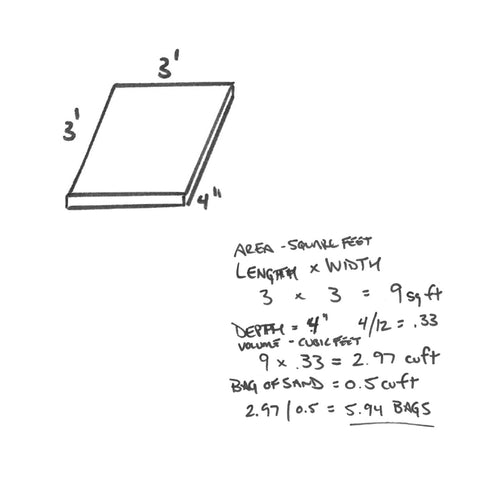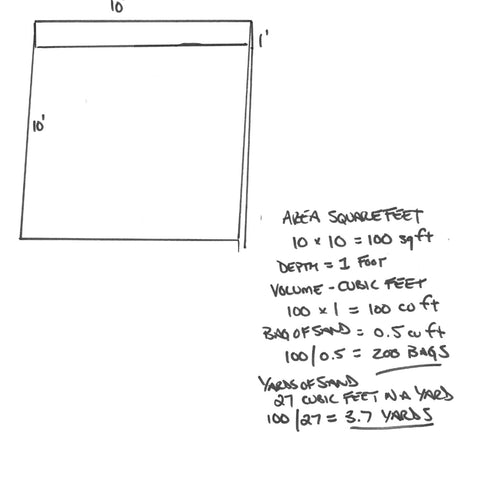# Sandbox Calculator

The Big Dig can be used in any size sandbox. While The Big Dig can dig to a total depth of 15", it can be used and enjoyed in as little as 3" of sand. The Big Dig can also reach 33" when the boom and stick (arm) are completely extended. If you have a standard 4' x 4' sandbox, a common method used by children is to set up The Big Dig outside of the sandbox and Dig In! Do you already have a sandbox or are you looking to get a new one? Use the calculator below to see how many bags of playsand you'll need to get digging.

Sandbox CalculationsFirst find the area of the sandbox in feet
3 feet long by 3 feet wide

Calculate the total area in Square Feet by multiplying Length x Width
Length x Width = Square Feet
3 x 3 = 9 square feet

Find the volume of the sandbox by finding the depth and convert to feet (inches divided by 12)
Depth ÷ 12
4 ÷ 12 = 0.33 cubic feet

Calculate the cubic feet of the sandbox my multiplying Area by depth
Area x Depth (in feet) = Cubic Feet
16 x 0.33 = 2.97 cubic feet

Find the cubic feet in a bag of playsand
Quikrete Playsand is 0.5 cubic feet

Calculate the number of bags needed by dividing the total cubic feet by the amount of cubic feet per bag of sand.
Cubic Feet ÷ Cubic Feet per bag = Number of bags
2.97 ÷ 0.5 = 5.94 bags of sandFor larger sandboxes (or sandpits) you may need to have your local supply yard deliver sand to your house. Our sandpit at headquarters is 10 feet x 10 feet x 1 foot deep.

To calculate the yards of sand needed, divide cubic feet of the sandpit by 27 (27 cubic feet in 1 yard)
Area ÷ Cubic Feet in a yard = Number of Yards
100 ÷ 27 = 3.7 yards

Our sandpit is 100 cubic feet and would need 200 bags of sand or 3.7 yards.